# 简介

java中和hash相关并且常用的有两个类hashTable和hashMap,两个类的底层存储都是数组，这个数组不是普通的数组，而是被称为散列表的东西。

# 数组和散列表

## hash的问题

Q（n）= 365/365×364/365×363/365×…×（365-n + 1）/ 365，即第一人的生日可以是365天中的任何一天，第二人的生日可以是除第一人的生日之外的任何365天，等等。

# 线性探测

h（v）//基地址
（h（v）+ 1 * 1）％M //第一个探测步骤，如果发生碰撞
（h（v）+ 2 * 1）％M //第二次探测步骤，如果仍有碰撞
（h（v）+ 3 * 1）％M //第三次探测步骤，如果仍有冲突

（h（v）+ k * 1）％M //第k个探测步骤等…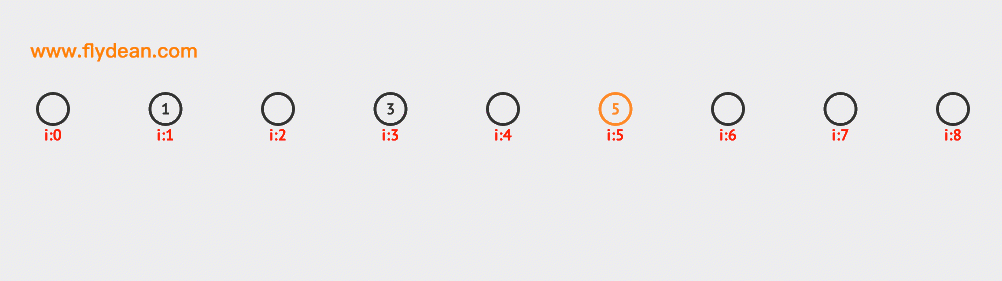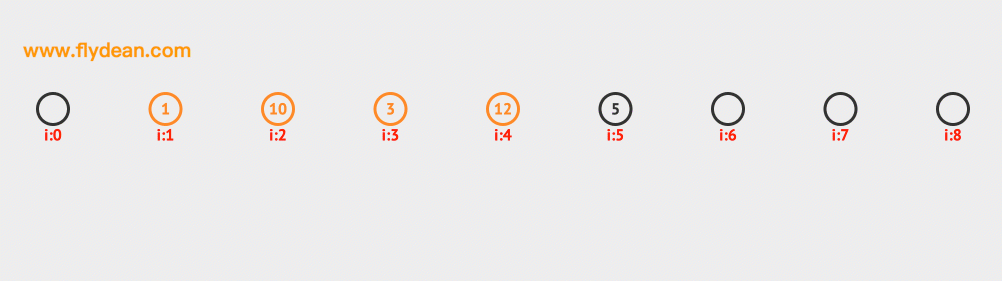``````    //插入节点
void insertNode(int key, int value)
{
HashNode temp = new HashNode(key, value);

//获取key的hashcode
int hashIndex = hashCode(key);

//find next free space
while(hashNodes[hashIndex] != null && hashNodes[hashIndex].key != key
&& hashNodes[hashIndex].key != -1)
{
hashIndex++;
hashIndex %= capacity;
}
//插入新节点，size+1
if(hashNodes[hashIndex] == null || hashNodes[hashIndex].key == -1) {
size++;
}
//将新节点插入数组
hashNodes[hashIndex] = temp;
}
``````

# 二次探测

h（v）//基地址
（h（v）+ 1 * 1）％M //第一个探测步骤，如果发生碰撞
（h（v）+ 2 * 2）％M //第2次探测步骤，如果仍有冲突
（h（v）+ 3 * 3）％M //第三次探测步骤，如果仍有冲突

（h（v）+ k * k）％M //第k个探测步骤等…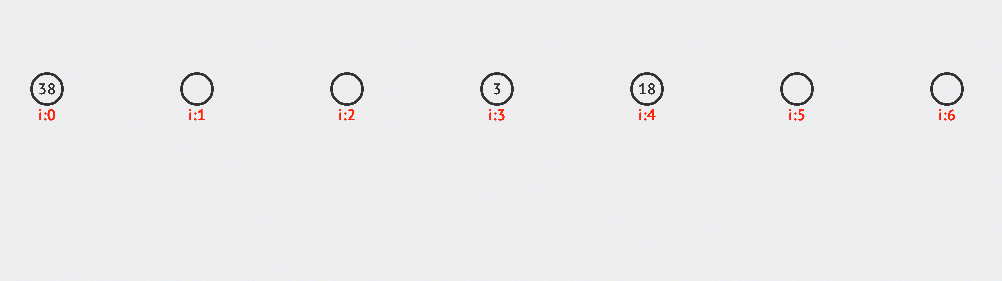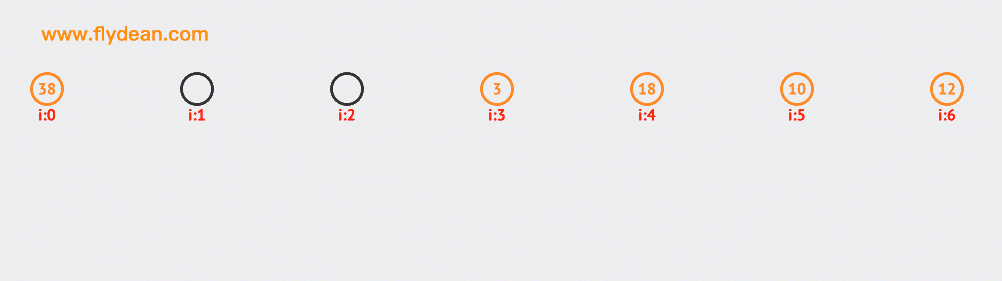``````    //插入节点
void insertNode(int key, int value)
{
HashNode temp = new HashNode(key, value);

//获取key的hashcode
int hashIndex = hashCode(key);

//find next free space
int i=1;
while(hashNodes[hashIndex] != null && hashNodes[hashIndex].key != key
&& hashNodes[hashIndex].key != -1)
{
hashIndex=hashIndex+i*i;
hashIndex %= capacity;
i++;
}

//插入新节点，size+1
if(hashNodes[hashIndex] == null || hashNodes[hashIndex].key == -1) {
size++;
}
//将新节点插入数组
hashNodes[hashIndex] = temp;
}
``````

# 双倍散列

h（v）//基地址
（h（v）+ 1 * h2（v））％M //第一个探测步骤，如果有碰撞
（h（v）+ 2 * h2（v））％M //第2次探测步骤，如果仍有冲突
（h（v）+ 3 * h2（v））％M //第三次探测步骤，如果仍有冲突

（h（v）+ k * h2（v））％M //第k个探测步骤等…

``````    //插入节点
void insertNode(int key, int value)
{
HashNode temp = new HashNode(key, value);

//获取key的hashcode
int hashIndex = hash1(key);

//find next free space
int i=1;
while(hashNodes[hashIndex] != null && hashNodes[hashIndex].key != key
&& hashNodes[hashIndex].key != -1)
{
hashIndex=hashIndex+i*hash2(key);
hashIndex %= capacity;
i++;
}

//插入新节点，size+1
if(hashNodes[hashIndex] == null || hashNodes[hashIndex].key == -1) {
size++;
}
//将新节点插入数组
hashNodes[hashIndex] = temp;
}
``````

# 分离链接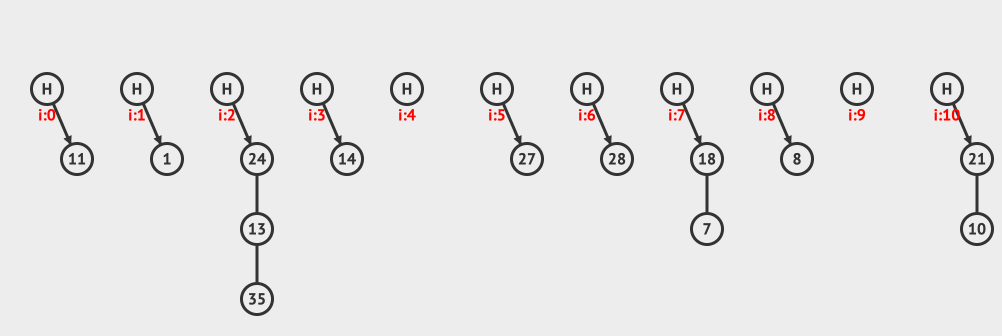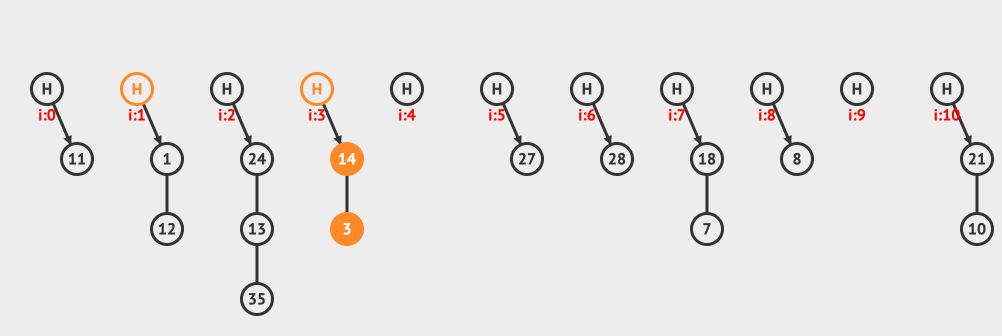`````` //添加元素
{

int index=hashCode(key);
{
size++;
}
else
{
{
{
size++;
break;
}
}
{
size++;
}
}
//动态扩容
if((1.0*size)/capacity>0.7)
{
HashNode[] tmp=hashNodes;
hashNodes=new HashNode[capacity*2];
capacity=2*capacity;
{
{
}
}
}
``````

learn-algorithm